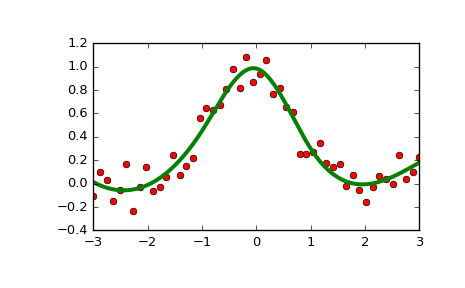# scipy.interpolate.LSQUnivariateSpline¶

class scipy.interpolate.LSQUnivariateSpline(x, y, t, w=None, bbox=[None, None], k=3, ext=0, check_finite=False)[source]

One-dimensional spline with explicit internal knots.

Fits a spline y = spl(x) of degree k to the provided x, y data. t specifies the internal knots of the spline

Parameters: x : (N,) array_like Input dimension of data points – must be increasing y : (N,) array_like Input dimension of data points t : (M,) array_like interior knots of the spline. Must be in ascending order and: bbox < t < ... < t[-1] < bbox[-1]  w : (N,) array_like, optional weights for spline fitting. Must be positive. If None (default), weights are all equal. bbox : (2,) array_like, optional 2-sequence specifying the boundary of the approximation interval. If None (default), bbox = [x, x[-1]]. k : int, optional Degree of the smoothing spline. Must be 1 <= k <= 5. Default is k=3, a cubic spline. ext : int or str, optional Controls the extrapolation mode for elements not in the interval defined by the knot sequence. if ext=0 or ‘extrapolate’, return the extrapolated value. if ext=1 or ‘zeros’, return 0 if ext=2 or ‘raise’, raise a ValueError if ext=3 of ‘const’, return the boundary value. The default value is 0. check_finite : bool, optional Whether to check that the input arrays contain only finite numbers. Disabling may give a performance gain, but may result in problems (crashes, non-termination or non-sensical results) if the inputs do contain infinities or NaNs. Default is False. ValueError If the interior knots do not satisfy the Schoenberg-Whitney conditions

UnivariateSpline
Superclass – knots are specified by setting a smoothing condition
InterpolatedUnivariateSpline
spline passing through all points
splrep
An older, non object-oriented wrapping of FITPACK
BivariateSpline
A similar class for two-dimensional spline interpolation

Notes

The number of data points must be larger than the spline degree k.

Knots t must satisfy the Schoenberg-Whitney conditions, i.e., there must be a subset of data points x[j] such that t[j] < x[j] < t[j+k+1], for j=0, 1,...,n-k-2.

Examples

>>> from scipy.interpolate import LSQUnivariateSpline, UnivariateSpline
>>> import matplotlib.pyplot as plt
>>> x = np.linspace(-3, 3, 50)
>>> y = np.exp(-x**2) + 0.1 * np.random.randn(50)


Fit a smoothing spline with a pre-defined internal knots:

>>> t = [-1, 0, 1]
>>> spl = LSQUnivariateSpline(x, y, t)

>>> xs = np.linspace(-3, 3, 1000)
>>> plt.plot(x, y, 'ro', ms=5)
>>> plt.plot(xs, spl(xs), 'g-', lw=3)
>>> plt.show()Check the knot vector:

>>> spl.get_knots()
array([-3., -1., 0., 1., 3.])


Constructing lsq spline using the knots from another spline:

>>> x = np.arange(10)
>>> s = UnivariateSpline(x, x, s=0)
>>> s.get_knots()
array([ 0.,  2.,  3.,  4.,  5.,  6.,  7.,  9.])
>>> knt = s.get_knots()
>>> s1 = LSQUnivariateSpline(x, x, knt[1:-1])    # Chop 1st and last knot
>>> s1.get_knots()
array([ 0.,  2.,  3.,  4.,  5.,  6.,  7.,  9.])


Methods

 __call__(x[, nu, ext]) Evaluate spline (or its nu-th derivative) at positions x. antiderivative([n]) Construct a new spline representing the antiderivative of this spline. derivative([n]) Construct a new spline representing the derivative of this spline. derivatives(x) Return all derivatives of the spline at the point x. get_coeffs() Return spline coefficients. get_knots() Return positions of interior knots of the spline. get_residual() Return weighted sum of squared residuals of the spline approximation. integral(a, b) Return definite integral of the spline between two given points. roots() Return the zeros of the spline. set_smoothing_factor(s) Continue spline computation with the given smoothing factor s and with the knots found at the last call.